﻿ A Class of Irrational Linear Multistep Block Method for the Direct Numerical Solution of Third Order Ordinary Differential EquationsPublications are Open
Access in this journal
Article Versions
Export Article
• Normal Style
• MLA Style
• APA Style
• Chicago Style
Research Article
Open Access Peer-reviewed

### A Class of Irrational Linear Multistep Block Method for the Direct Numerical Solution of Third Order Ordinary Differential Equations

Bamikole Gbenga Ogunware, Ezekiel Olaoluwa Omole
Turkish Journal of Analysis and Number Theory. 2020, 8(2), 21-27. DOI: 10.12691/tjant-8-2-1
Received May 31, 2020; Revised June 23, 2020; Accepted July 02, 2020

### Abstract

This work considers the direct solution of general third order ordinary differential equation by three-step irrational linear multistep method. This method is derived using collocation and interpolation techniques. An irrational three-step method is developed. Taylor series and block methods are used to generate the independent solution at selected points. The properties of the method were also determined. The developed method was applied on general third order ordinary differential equations. And the performance of the numerical results of the method compared favourably with the results of existing authors in the recent literature to test its accuracy and stability.

### 1. Introduction

The numerical solution of general third order initial value problems of the form: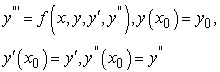(1)

is considered. Where f is continuous and satisfies Lipchitz’s condition that guarantees the uniqueness and existence of a solution.

Problems in the form (1) have wide application in engineering, social science, physical science, etc. Very often, these problems do not have an analytical solution, and this has necessitated the use of numerical method to approximate their solutions. Previously, equation (1) is solved by reducing it to its equivalent system of first order ordinary differential equations and thereafter appropriate numerical method for first order would be applied to solve the systems. However, it is shown in 1, 2, that this reduction approach has serious problems which include consumption of human effort, computational burden and non-economization of computer time.

Linear multistep methods for solving equation (1) directly have been proposed by some researchers such as 3, 4, 5, 6. A P-stable linear multistep method for direct solution of equation (1) was developed by 7 which was implemented in predictor-corrector mode. In order to cater for the setbacks encountered in the reduction approach and also bring about improvement on numerical method accuracy 8, 9, 10 developed block methods for solving higher order ordinary differential equations directly in which the accuracy is better than when it is reduced to system of first order ordinary differential equations.

Also, various authors such as 11, 12, 13, 14, 15, 16 developed hybrid methods. This method, while retaining certain characteristics of the continuous linear multistep method, share with Runge-Kutta Methods the property of utilizing data at other points, other than the step point. Block method was found to be efficient in handling equation (1). It is also cost effective.

The use of irrational linear multistep method is uncommon in numerical analysis literatures; hence this article discusses the derivation and application of a three-step block method with one irrational point for the solution of equation (1) directly.

### 2. Derivation of the Method (Scheme)

In developing this method, power series of the form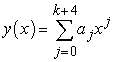(2)

is considered as the approximate solution to equation(1), where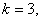equation (3) is derived by differentiating equation(2) three times to give.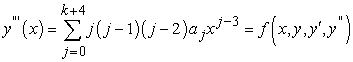(3)

Interpolating (2) at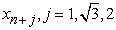and collocating equation (3) at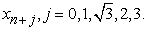These equations are then combined to give a nonlinear system of equations of the form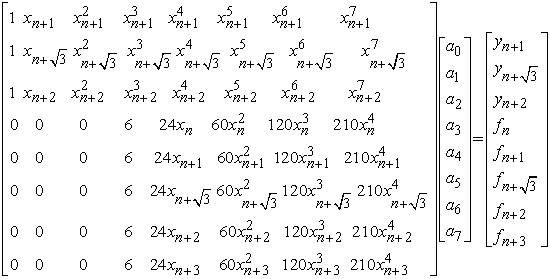(4)

Gaussian elimination technique is used in finding the values of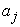’s in equation (4) which are then substituted into equation (2) to produce a continuous implicit scheme of the form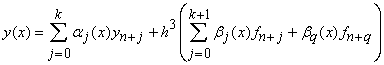(5)

Where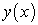is the numerical solution of the initial value problem and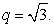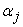and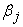are both constant.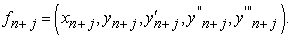(6)

Using the transformation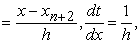we obtain the continuous scheme below.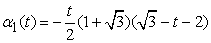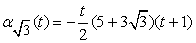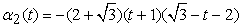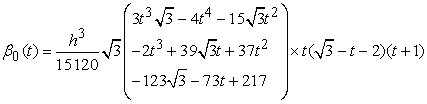(7)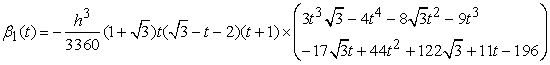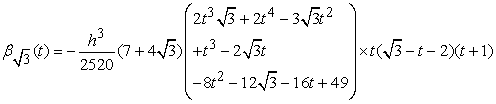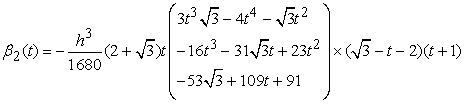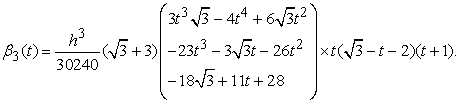Evaluating the above continuous method at the end point, gives the discrete scheme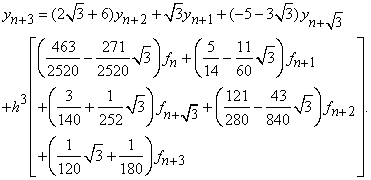(8)
2.1. Derivation of the Block

Normalizing the combination of the discrete scheme with the evaluation of the first and second derivatives of the continuous method at all the points yield the block below.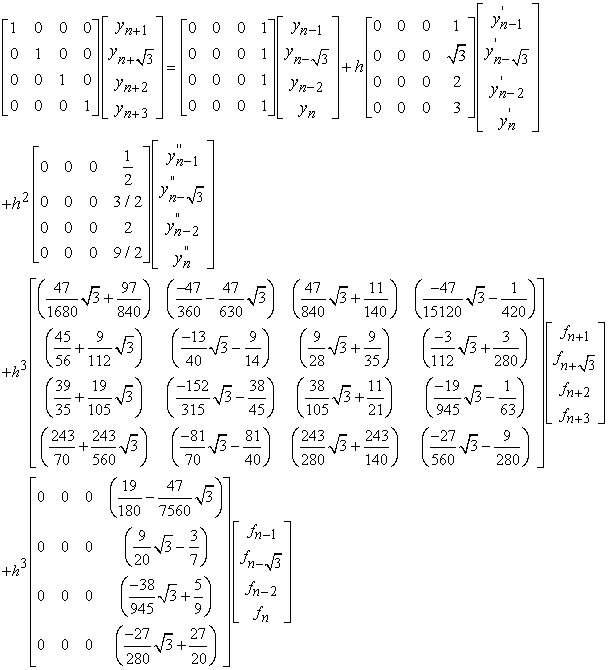(9)

Writing out the block explicitly gives the following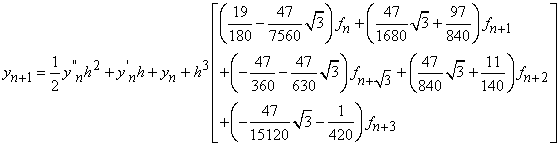(10)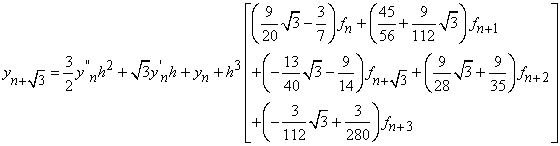(11)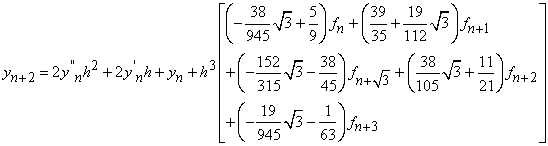(12)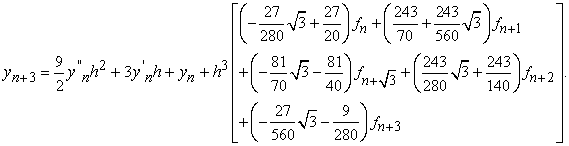(13)

### 3. Analysis of the Basic Properties of the Block

3.1. Order and Error Constant of the Block

A block linear multistep method is said to be of order p if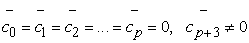Thus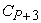is the error constant. See 7

For our three step irrational method, expanding the block in Taylor series expansion gives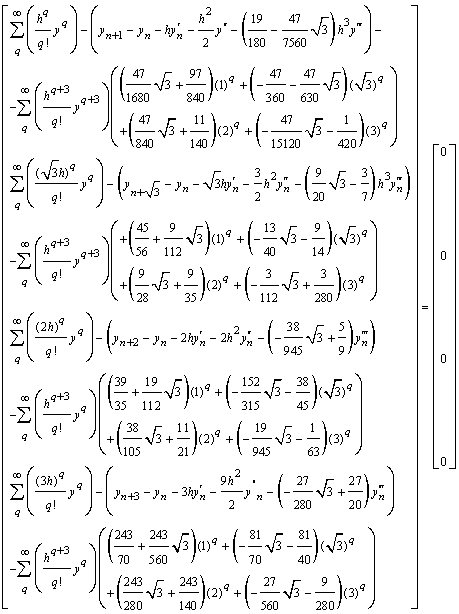(14)

Hence, the irrational block is of order 5, with error constant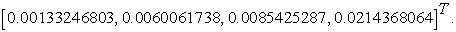3.2. Zero Stability of the Block Method

Given the general form of block method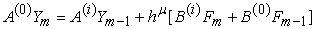A block method is said to be zero stable, if the roots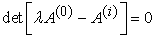of the first characteristic polynomial satisfyand for the roots with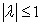, the multiplicity must not exceed the order of the differential equation. See 7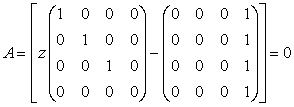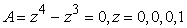Hence the block is zero stable.

3.3. Region of Absolute Stability

The Stability nature of the method is found in the Spirit of 7 and 4 as shown below

• Figure 1. Showing the region of absolute stability of the irrational method
3.4. Convergence

Theorem 1: Convergence 17

The necessary and sufficient condition for a linear multistep method to be convergent is for it to be consistent and zero stable. From the theorem above, the irrational block method is convergent.

### 4. Numerical Experiments

Here, the performance of the new irrational method is examined on some test examples. The results obtained from the test examples are shown in tabular and graphical form. We used MATLAB codes for the computational purposes.

Example 1: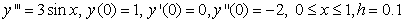Exact solution: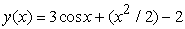Source: 18 and 10

Example 2: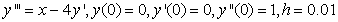Exact solution: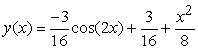Source: 19 and 20

• Figure 2. Showing the comparison of the result of problem 1 with  and 
• Figure 3. Showing the comparison of the result of problem 2 with  and 

### 5. Conclusion

From the two test problems solved by the new irrational method, we can conclude that the new method is effective in handling third order ordinary differential equations initial value problems. This fact is clearly seen from the accuracy of the results presented in the Table 1 and Table 2 and graphs in Figure 2 and Figure 3 above.

### References

  Awoyemi, D. O., A class of continuous method for general second order initial value problems in ordinary differential equations. International journal of computer mathematics, vol. 72 29-39, 1999. In article View Article  Awoyemi, D. O., A new sixth order algorithms for General Second order ordinary differential equation. Inter. J. Computer Math., 77 117-124, 2001. In article View Article  Brugnano L. and Trigiante, D., Solving Differential Problems by Multistep Initial and Boundary Value Methods, Gordon and Breach Science Publishers, Amsterdam, pp. 280-299,. 1998. In article  Kayode, S.J.,An improved Numerov method for direct solution of general second order initial value problems of ordinary differential equations.Proceeding of National Mathematical Centre, 2005. In article  Adesanya, A. O. Anake, T. A and Oghoyon, G. J., Continuous Implicit Method for the solution of General Second order ordinary differential equation. Journal of Nigerian Association of Mathematical Physics, 40,71-78, 2009. In article  Olabode, B. T., An accurate scheme by block method for third order ordinary differential equations. The Pacific Journal of Science and Technology 10(1), 136 .142, 2009. In article  Awoyemi, D. O., A p-stable linear multistep method for solving third order ordinary differential equation, International Journal of Computer Mathematics 80(8), 85-91, 2003. In article View Article  Omar, Z. and Suleiman, M., Parallel R-Point implicit block method for solving higher order ordinary differential equation directly, Journal of ICT. 3(1), 53-66, 2003. In article  Badmus, A. M and Yahaya, Y. A., An accurate uniform order 6 for the direct solution of general second order ordinary differential equations. The Pacific of Science and Technology. 2009;10 (2):248-253, 2009. In article  Ogunware, B. G.,Omole, E. O., and Olanegan, O.O., Hybrid and non-hybrid implicit schemes for solving third order ODEs using block method as predictors. Mathematical Theory and Modeling. 2015;5(3):10-25, 2015. In article  Kayode, S. J and Adeyeye, O., A 3-Step Hybrid Method for the Direct Solution of Second Order Initial Value Problems. Australian Journal of Basic and Applied Sciences, 5 (12): 2121-2126, 2011. In article  Ogunware, B.G., Numerical Solution of Third Order Ordinary Differential Equations: Lambert Academic Publishing (LAP), 2015. In article  Olanegan, O.O., Awoyemi, D.O., Ogunware, B. G and Obarhua, F.O., Continuous double-hybrid point method for the solution of second order ordinary differential equations. IJAST. 5(2):549-562, 2015. In article  Omar, Z and Kuboye, J. O., Computation of an Accurate Implicit Block Method for Solving Third Order. Global journal of pure and applied mathematics, ISSN 0973-1768 Volume 11, Number 1, pp. 177-186, 2015. In article View Article  Omar, Z. and Abdelrahim, R., New Uniform Order Single Step Hybrid Block Method for Solving Second Order Ordinary Differential Equations. International Journal of Applied Engineering Research ISSN 0973-4562 Volume 11, Number 4 pp2402-2406. In article  Omole, E. O. and Ogunware, B.G.,3- Point Single Hybrid Block Method (3PSHBM) for Direct Solution of General Second Order Initial Value Problem of Ordinary Differential Equations. Journal of Scientific Research & Reports 20(3): 1-11; Article no.JSRR.19862ISSN: 2320-0227, 2018. In article View Article  Lambert, J. D., Computational methods in ordinary differential equation, John Wiley & Sons Inc. New York, 1973. In article  Olabode, B. T., Some linear multistep methods for special and general third order initial value problems of ordinary differential equation, PhD Thesis, Federal University of Technology, Akure, 2007. In article  Adoghe,L. O., Ogunware, B.G and Omole, E. O., A family of symmetric implicit higher order methods for the solution of third order initial value problems in ordinary differential equations. Theoretical Mathematics & Applications, vol. 6, no. 3, 67-84 ISSN: 1792-9687 (print), 1792-9709 (online) Science press Ltd, 2016. In article  Adesanya, A. O., Block methods for direct solution of general higher order initial value problems of ordinary differential equations, PhD Thesis, Federal University of Technology, Akure.(Unpublished), 2011. In article

Published with license by Science and Education Publishing, Copyright © 2020 Bamikole Gbenga Ogunware and Ezekiel Olaoluwa OmoleThis work is licensed under a Creative Commons Attribution 4.0 International License. To view a copy of this license, visit http://creativecommons.org/licenses/by/4.0/